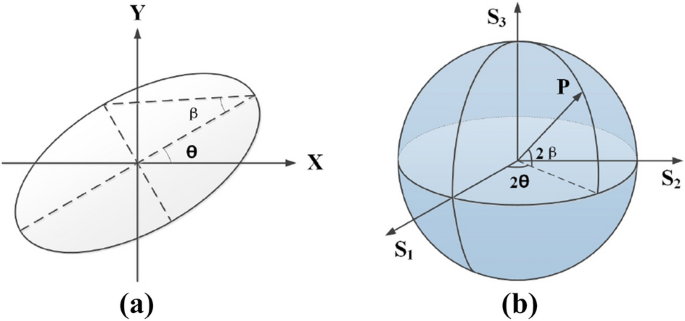# Unitary transformation for Poincaré beams on different parts of Poincaré sphereIn this research, the common focus of a 4f system was set on the focal image plane (FIP) of the two cascade biaxial crystals with the length of (L_1) and (L_2), as shown in Fig. 1, resulting in the Unitary transformation of light field. The optic axis of the two biaxial crystals, which the crystal are rotated around, are parallel with each other and the principal refractive indices (n_1<n_2<n_3).

According to the previous studies by Berry and Phelan25,28, the Fourier transform ({mathbf{a}}({mathbf{P}},z)) of the electric field ({{mathbf{E}}_{{mathbf{CR}}}}({mathbf{r}},Z)), which is transformed by conical diffraction at the plane with the length of Z from FIP can be expressed as27

begin{aligned} {mathbf{a}}({mathbf{P}},z) = exp left( { – frac{1}{2}ik{P^2}Z} right) cdot {mathbf{T}} cdot {mathbf{a}}({mathbf{P}},0) end{aligned}

(1)

({mathbf{P}}) is the dimensionless transverse wavevector of plane waves ({mathbf{k}}). ({mathbf{a}}({mathbf{P}},0)) is the Fourier transform of the input electric field ({{mathbf{mathrm{E}}}_{in}}). k is the wavenumber in crystal satisfying (k = {n_2}{k_0}).

The transformation matrix T represents the manipulation of the beam passing through cascade biaxial crystals.

begin{aligned} {mathbf{T}} = {mathbf{R}}(alpha ){mathbf{C}}(P,phi – alpha ,{R_{02}}){mathbf{R}}( – alpha ){mathbf{C}}(P,phi ,{R_{01}}) end{aligned}

(2)

where, ({mathbf{R}}left( alpha right))is the rotation matrix satisfying ({mathbf{R}}left( alpha right) = left[ {begin{array}{*{20}{c}} {cos alpha }&{}{ – sin alpha }\ {sin alpha }&{}{cos alpha } end{array}} right]). The matrix ({mathbf{C}}left( {P,phi ,{R_0}} right) = cos left( {kP{R_0}} right) {mathbf{I}} – isin left( {kP{R_0}} right) {mathbf{M}}left( phi right)) is the transformation matrix for single biaxial crystal with the length of L. ({mathbf{r}} = left( {r,phi } right)) is the position at the transverse plane in cylinder coordinates. The matrix ({mathbf{M}}left( phi right)) equals (left[ {begin{array}{*{20}{c}} {cos phi }&{}{sin phi }\ {sin phi }&{}{ – cos phi } end{array}} right]) and ({mathbf{I}}) is the identity matrix by (2 times 2). ({R_0} = left( sqrt{left( {{n_3} – {n_2}} right) left( {{n_2} – {n_1}} right) } /{n_2}right) L) is the radius of the refracted ring beam beyond the crystal.

Through the second lens, the light field in the output plane of our system can be expressed as27,

begin{aligned} {{mathbf {mathrm{E}}}_{out}}({mathbf {r}}) = {mathbf {T}} cdot {{mathbf {mathrm{E}}}_{in}}({mathbf {r}}) = {E_{in}}{mathbf {T}} cdot left[ {begin{array}{*{20}{c}} {{e_x}} \ {{e_y}} end{array}} right] end{aligned}

(3)

({left[ {{{e_x}}quad {{e_y}}} right] ^T}) represents the SoP of input beam, for instance, ({left[ {{{1}}quad {{i}}} right] ^T}) and ({left[ {{{0}}quad {{1}}} right] ^T}) denote the left-handed circular and y-linear polarization, respectively.

The transformation matrix ({mathbf {T}}) has been demonstrated to be a Unitary matrix satisfying ({{mathbf {T}}^*} cdot {mathbf {T}} = {mathbf {mathrm{I}}}) with arbitrary rotation angle (alpha), as shown in Fig. 1b.

Especially for the case that the cascade crystals have equal length as well as the equal (R_0). The elements of matrix T expressed as (T_{11}), (T_{12}), (T_{21}) and (T_{22}) can be derived as

begin{aligned} begin{array}{l} {T_{11}} = [(1 + cos (2kP{R_0})) – cos (alpha )(1 – cos(2kP{R_0})) – i(cos (phi ) + cos (alpha + phi ))sin (2kP{R_0})]/2\ {T_{12}} = [sin (alpha )(1 – cos (2kP{R_0})) – i(sin (phi ) + sin (alpha + phi ))sin (2kP{R_0})]/2\ {T_{21}} = [ – sin (alpha )(1 – cos (2kP{R_0})) – i(sin (phi ) + sin (alpha + phi ))sin (2kP{R_0})]/2\ {T_{22}} = [(1mathrm{{ + }}cos (2kP{R_0})) – cos (alpha )(1 – cos (2kP{R_0})) + i(cos (phi ) + cos (alpha + phi ))sin (2kP{R_0})]/2 end{array} end{aligned}

(4)

Therefore, the Stokes parameters ({S_0},{S_1},{S_2},{S_3}) could be calculated from these intensity distributions by the formula as follows.

begin{aligned} begin{aligned} {S_0} =,&{left| {{E_x}} right| ^2} + {left| {{E_y}} right| ^2}{text { = }}{I_{0^circ }} + {I_{90^circ }} \ {S_1} =,&{left| {{E_x}} right| ^2} – {left| {{E_y}} right| ^2} = {I_{0^circ }} – {I_{90^circ }} \ {S_2} =,&2{text {Re}} left[ {{E_x}^*{E_y}} right] = {I_{45^circ }} – {I_{ – 45^circ }} \ {S_3} =,&2{text {Im}} left[ {{E_x}^*{E_y}} right] = {I_R} – {I_L} \ end{aligned} end{aligned}

(5)

When the incident beam is in right-handed circular polarization, the Stokes parameters representing the polarization of the beam can be subsequently obtained as follows

begin{aligned} begin{array}{l} {S_0} = {I_{mathrm{{in}}}}\ {S_1} = {I_{mathrm{{in}}}}sin (2kP{R_0})[ – sin (2alpha + phi ) – sin (alpha + phi ) + (sin (alpha + phi ) + sin (phi )/2 + sin (2alpha + phi )/2)(1 + cos (2kP{R_0}))]\ {S_2} = {I_{mathrm{{in}}}}sin (2kP{R_0})[cos (2alpha + phi ) + cos (alpha + phi ) – (cos (alpha – phi ) + cos (phi )/2 + cos (2alpha + phi )/2)(1 + cos (2kP{R_0}))]\ {S_3} = {I_{mathrm{{in}}}}[1 – sin {(2kP{R_0})^2}(1 + cos(alpha ))] end{array} end{aligned}

(6)

As a result, the azimuth angle (theta) and the ellipticity angle (beta) of the polarization ellipse, which is also a useful way to visualize polarization, can be confirmed uniquely by means of measuring the Stokes parameters, as shown in Fig. 2.

begin{aligned} begin{array}{l} 2theta = arctan (frac{{{S_2}}}{{{S_1}}}) =arctan left[ frac{{ – cos (alpha + phi ) – cos (2alpha + phi ) + 2cos (alpha + phi )(1 + cos (alpha )) cos {{(kP{R_0})}^2}}}{{ – cos (alpha + phi ) – cos (2alpha + phi ) + 2sin (alpha + phi )(1 + cos (alpha ))cos {{(kP{R_0})}^2}}}right] \ 2beta = arcsin left( frac{{{S_3}}}{sqrt{{S_1}^2+{S_2}^2}}right) =arcsin (1 – sin {(2kP{R_0})^2}(1 + cos (alpha ))) end{array} end{aligned}

(7)Figure 2

In which, the range of ellipticity angle (beta in [alpha /2 – pi /4,pi /4]) reveals the relation of the proportion of SoPs projected on the Poincaré sphere and the rotation angle (alpha) of second crystal. The effects are similar for the cases that the incident beam is in different polarization.